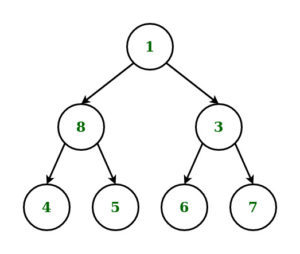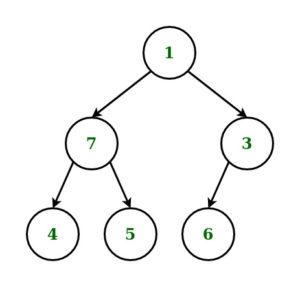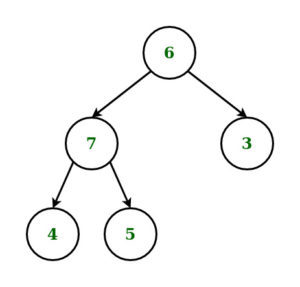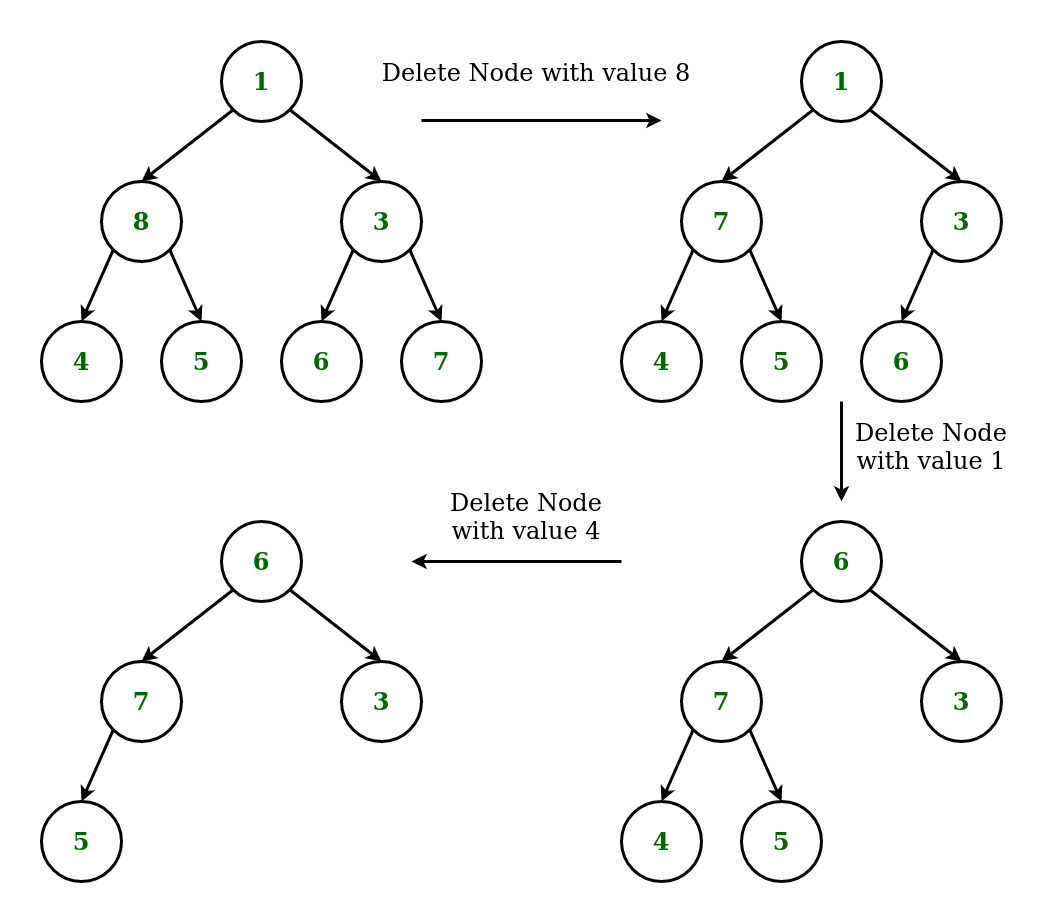# Deletion of a given node K in a Binary Tree using Level Order Traversal

• Difficulty Level : Medium
• Last Updated : 11 May, 2022

Given a binary tree and a node K, the task is to delete the node K from it by making sure that tree shrinks from the bottom (i.e. the deleted node is replaced by bottom-most and rightmost node) using Level Order Traversal.

Examples:

Input: K = 8, Tree =Output:Explanation:

Input: K = 1, Tree =Output:Approach:

• Start searching from the root, the address of node which is to be deleted by traversing in level order-wise.
• Continue Traversing Tree to find the deepest and rightmost node in level order wise to find the deepest and the rightmost node.
• If the node to delete is different from rightmost deepest node, then replace the node to be deleted with rightmost deepest node and delete the later node
• If the node to delete is same as rightmost deepest node, then simply delete the node.

For example: Consider the Example 1 from aboveBelow is the implementation of the above approach.

## C++

 `// C++ implementation to delete a node` `// in Binary Tree using Level Order Traversal`   `#include ` `using` `namespace` `std;`   `// Structure of Binary Tree` `struct` `node {` `    ``int` `data;` `    ``node* left;` `    ``node* right;` `};`   `// Function to create new node` `// of a Binary Tree.` `node* newnode(``int` `d)` `{` `    ``node* temp = ``new` `node;` `    ``temp->data = d;` `    ``temp->left = temp->right = NULL;` `    ``return` `temp;` `}`   `// Function for Level Order` `// Traversal of Binary Tree` `void` `levelorder(node* curr)` `{`   `    ``// Queue to store the nodes` `    ``// in a level` `    ``queue q;` `    ``if` `(curr)` `        ``q.push(curr);`   `    ``// Loop to Traverse the Binary` `    ``// Tree in Level Order` `    ``while` `(!q.empty()) {` `        ``curr = q.front();` `        ``q.pop();` `        ``cout << curr->data << ``" "``;` `        ``if` `(curr->left)` `            ``q.push(curr->left);` `        ``if` `(curr->right)` `            ``q.push(curr->right);` `    ``}` `}`   `// Function to Delete the deepest` `// right-most node of the Binary Tree` `void` `deletedeepest(node* root,` `                ``node* temp)` `{` `    ``queue q;` `    ``q.push(root);`   `    ``// Loop to Traverse Binary Tree` `    ``// in level order and delete Node` `    ``while` `(!q.empty()) {` `        ``node* T = q.front();` `        ``q.pop();` `        ``if` `(T->right == temp) {` `            ``T->right = NULL;` `            ``delete` `(temp);` `            ``return``;` `        ``}` `        ``else` `            ``q.push(T->right);` `        ``if` `(T->left == temp) {` `            ``T->left = NULL;` `            ``delete` `(temp);` `            ``return``;` `        ``}` `        ``else` `            ``q.push(T->left);` `    ``}` `}`   `// Function to Delete Node` `// in Binary Tree` `node* deletenode(node* root, ``int` `data)` `{` `    ``if` `(root == NULL)` `        ``return` `NULL;`   `    ``// Condition if the Root node` `    ``// is a leaf node.` `    ``if` `(root->left == NULL` `        ``&& root->right == NULL) {` `        ``if` `(root->data == data) {` `            ``return` `NULL;` `        ``}` `        ``else` `            ``return` `root;` `    ``}` `    ``queue q;` `    ``q.push(root);` `    ``node* temp = NULL;` `    ``node* nodetodelete = NULL;`   `    ``// Loop to Traverse Tree in` `    ``// Level Order and find the` `    ``// Rightmost Deepest Node of the` `    ``// Binary Tree and Node to be Deleted` `    ``while` `(!q.empty()) {` `        ``temp = q.front();` `        ``q.pop();` `        ``if` `(temp->data == data) {` `            ``nodetodelete = temp;` `        ``}` `        ``if` `(temp->left) {` `            ``q.push(temp->left);` `        ``}` `        ``if` `(temp->right) {` `            ``q.push(temp->right);` `        ``}` `    ``}`   `    ``// if node to be deleted is not found` `    ``if` `(nodetodelete != NULL) {`   `        ``// Condition to check if node to delete` `        ``// is not same as node to replace` `        ``if` `(temp != nodetodelete) {` `            ``int` `t = temp->data;` `            ``deletedeepest(root, temp);` `            ``nodetodelete->data = t;` `        ``}`   `        ``// if node to delete is also` `        ``// rightmost deepest node` `        ``else` `{` `            ``deletedeepest(root, temp);` `        ``}` `    ``}` `    ``return` `root;` `}`   `// Driver Code` `int` `main()` `{`   `    ``// Construction of Tree` `    ``node* root = newnode(1);` `    ``root->left = newnode(8);` `    ``root->right = newnode(3);` `    ``root->left->left = newnode(4);` `    ``root->left->right = newnode(5);` `    ``root->right->left = newnode(6);` `    ``root->right->right = newnode(7);`   `    ``cout << ``"Original Tree: "``;` `    ``levelorder(root);`   `    ``// Deleting node with key 8` `    ``root = deletenode(root, 8);` `    ``cout << endl;` `    ``cout << ``"Deleting node with key 8: "``;` `    ``levelorder(root);`   `    ``// Deleting node with key 1` `    ``root = deletenode(root, 1);` `    ``cout << endl;` `    ``cout << ``"Deleting node with key 1: "``;` `    ``levelorder(root);`   `    ``// Deleting node with key 4` `    ``root = deletenode(root, 4);` `    ``cout << endl;` `    ``cout << ``"Deleting node with key 4: "``;` `    ``levelorder(root);` `}`

## Java

 `// Java implementation to delete a node ` `// in Binary Tree using Level Order Traversal ` `import` `java.util.LinkedList;` `import` `java.util.Queue;`   `// Binary Tree ` `class` `TreeNode` `{` `    ``int` `data;` `    ``TreeNode left = ``null``;` `    ``TreeNode right = ``null``;` `    `  `    ``TreeNode(``int` `data)` `    ``{` `        ``this``.data = data;` `    ``}` `}`   `class` `BinaryTreeDeleteKNode{`   `public` `TreeNode insert(TreeNode root,` `                    ``int` `value)` `{` `    ``if` `(root == ``null``) ` `    ``{` `        ``root = ``new` `TreeNode(value);` `        ``return` `root;` `    ``}` `    `  `    ``// Do level order traversal and add ` `    ``// node to first null` `    ``Queue q = ``new` `LinkedList();` `    ``q.add(root);` `    `  `    ``while` `(!q.isEmpty())` `    ``{` `        ``TreeNode tn = q.remove();` `        ``if` `(tn.left != ``null``)` `        ``{` `            ``q.add(tn.left);` `        ``}` `        ``else` `        ``{` `            ``tn.left = ``new` `TreeNode(value);` `            ``return` `root;` `        ``}` `        ``if` `(tn.right != ``null``)` `        ``{` `            ``q.add(tn.right);` `        ``}` `        ``else` `        ``{` `            ``tn.right = ``new` `TreeNode(value);` `            ``return` `root;` `        ``}` `    ``}` `    ``return` `root;` `}`   `// Function for Level Order ` `// Traversal of Binary Tree ` `public` `void` `levelOrder(TreeNode root)` `{` `    ``if` `(root == ``null``)` `    ``{` `        ``System.out.println(``"Tree is empty!!"``);` `    ``}` `    `  `    ``// Queue to store the nodes ` `    ``// in a level ` `    ``Queue q = ``new` `LinkedList();` `    ``q.add(root);` `    `  `    ``// Loop to Traverse the Binary ` `    ``// Tree in Level Order ` `    ``while` `(!q.isEmpty())` `    ``{` `        ``TreeNode tn = q.remove();` `        ``System.out.print(tn.data + ``" "``);` `        `  `        ``if` `(tn.left != ``null``)` `            ``q.add(tn.left);` `        ``if` `(tn.right != ``null``)` `            ``q.add(tn.right);` `    ``}` `}`   `// Function to delete node node with value K` `public` `TreeNode deleteIn(TreeNode root, ``int` `k)` `{` `    ``if` `(root == ``null``) ` `        ``return` `root;` `    `  `    ``// Do level order traversal if node found ` `    ``// with the value k then select that node` `    ``// keep traversal till we find deepest ` `    ``// node with empty value keep track of ` `    ``// the parent node of the deepest node` `    ``TreeNode searchedNode = ``null``;` `    ``TreeNode tn = ``null``;` `    `  `    ``Queue q = ``new` `LinkedList();` `    ``TreeNode deepestNodeParent = ``null``;` `    ``q.add(root);` `    `  `    ``while` `(!q.isEmpty())` `    ``{` `        ``boolean` `isParent = ``false``;` `        ``tn = q.remove();` `        `  `        ``if` `(searchedNode == ``null` `&& tn.data == k)` `        ``{` `            ``searchedNode = tn;` `        ``}` `        ``if` `(tn.left != ``null``)` `        ``{` `            ``q.add(tn.left);` `            ``isParent = ``true``;` `        ``}` `        ``if` `(tn.right != ``null``)` `        ``{` `            ``q.add(tn.right);` `            ``isParent = ``true``;` `        ``}` `        ``if` `(isParent) deepestNodeParent = tn;` `    ``}`   `    ``if` `(searchedNode == ``null``)` `    ``{` `        ``System.out.print(``"Node with value '"` `+ k + ` `                        ``"' not exists."``);` `        ``return` `root;` `    ``}` `    ``searchedNode.data = tn.data;` `    ``if` `(deepestNodeParent != ``null` `&&` `        ``deepestNodeParent.left != ``null` `&&` `        ``deepestNodeParent.left.data == tn.data)` `    ``{` `    ``deepestNodeParent.left = ``null``;` `    ``}` `    ``else` `    ``{` `        ``deepestNodeParent.right = ``null``;` `    ``}` `    ``return` `root;` `}`   `// Driver code` `public` `static` `void` `main(String[] args)` `{` `    ``TreeNode node = ``null``;` `    ``BinaryTreeDeleteKNode binaryTreeDeleteKNode = ``new` `BinaryTreeDeleteKNode();` `    `  `    ``// Construction of Tree` `    ``node = binaryTreeDeleteKNode.insert(node, ``1``);` `    ``node = binaryTreeDeleteKNode.insert(node, ``8``);` `    ``node = binaryTreeDeleteKNode.insert(node, ``3``);` `    ``node = binaryTreeDeleteKNode.insert(node, ``4``);` `    ``node = binaryTreeDeleteKNode.insert(node, ``5``);` `    ``node = binaryTreeDeleteKNode.insert(node, ``6``);` `    ``node = binaryTreeDeleteKNode.insert(node, ``7``);` `    `  `    ``System.out.print(``"Original Tree: "``);` `    ``binaryTreeDeleteKNode.levelOrder(node);` `    `  `    ``// Deleting node with key 8` `    ``node = binaryTreeDeleteKNode.deleteIn(node,``8``);` `    ``System.out.print(``"\nDeleting node with key 8: "``);` `    ``binaryTreeDeleteKNode.levelOrder(node);` `    `  `    ``// Deleting node with key 1` `    ``node = binaryTreeDeleteKNode.deleteIn(node,``1``);` `    ``System.out.print(``"\nDeleting node with key 1: "``);` `    ``binaryTreeDeleteKNode.levelOrder(node);` `    `  `    ``// Deleting node with key 4` `    ``node = binaryTreeDeleteKNode.deleteIn(node,``4``);` `    ``System.out.print(``"\nDeleting node with key 4: "``);` `    ``binaryTreeDeleteKNode.levelOrder(node);` `}` `}`   `// This code is contributed by anshulgtbit91`

## Python3

 `# Python3 implementation to delete a node` `# in Binary Tree using Level Order Traversal` `from` `collections ``import` `deque`   `# Structure of Binary Tree` `class` `Node:` `    `  `    ``def` `__init__(``self``, x):` `        `  `        ``self``.data ``=` `x` `        ``self``.left ``=` `None` `        ``self``.right ``=` `None`   `# Function for Level Order` `# Traversal of Binary Tree` `def` `levelorder(curr):` `    `  `    ``# Queue to store the nodes` `    ``# in a level` `    ``q ``=` `deque()`   `    ``if` `(curr):` `        ``q.append(curr)`   `    ``# Loop to Traverse the Binary` `    ``# Tree in Level Order` `    ``while` `len``(q) > ``0``:` `        ``curr ``=` `q.popleft()` `        ``#q.pop()` `        ``print``(curr.data, end ``=` `" "``)` `        `  `        ``if` `(curr.left):` `            ``q.append(curr.left)` `        ``if` `(curr.right):` `            ``q.append(curr.right)`   `# Function to Delete the deepest` `# right-most node of the Binary Tree` `def` `deletedeepest(root, temp):` `    `  `    ``q ``=` `deque()` `    ``q.append(root)`   `    ``# Loop to Traverse Binary Tree` `    ``# in level order and delete Node` `    ``while` `(``len``(q) > ``0``):` `        ``T ``=` `q.popleft()` `        ``#q.pop()` `        `  `        ``if` `(T.right ``=``=` `temp):` `            ``T.right ``=` `None` `            ``#delete (temp)` `            ``return` `        ``else``:` `            ``q.append(T.right)` `            `  `        ``if` `(T.left ``=``=` `temp):` `            ``T.left ``=` `None` `            ``#delete (temp)` `            ``return` `        ``else``:` `            ``q.append(T.left)`   `# Function to Delete Node` `# in Binary Tree` `def` `deletenode(root, data):` `    `  `    ``if` `(root ``=``=` `None``):` `        ``return` `None`   `    ``# Condition if the Root node` `    ``# is a leaf node.` `    ``if` `(root.left ``=``=` `None` `and` `        ``root.right ``=``=` `None``):` `        ``if` `(root.data ``=``=` `data):` `            ``return` `None` `        ``else``:` `            ``return` `root`   `    ``q ``=` `deque()` `    ``q.append(root)` `    ``temp ``=` `None` `    ``nodetodelete ``=` `None`   `    ``# Loop to Traverse Tree in` `    ``# Level Order and find the` `    ``# Rightmost Deepest Node of the` `    ``# Binary Tree and Node to be Deleted` `    ``while` `(``len``(q) > ``0``):` `        ``temp ``=` `q.popleft()` `        ``#q.pop()` `        `  `        ``if` `(temp.data ``=``=` `data):` `            ``nodetodelete ``=` `temp` `        ``if` `(temp.left):` `            ``q.append(temp.left)` `        ``if` `(temp.right):` `            ``q.append(temp.right)`   `    ``# If node to be deleted is not found` `    ``if` `(nodetodelete !``=` `None``):` `        `  `        ``# Condition to check if node to delete` `        ``# is not same as node to replace` `        ``if` `(temp !``=` `nodetodelete):` `            ``t ``=` `temp.data` `            ``deletedeepest(root, temp)` `            ``nodetodelete.data ``=` `t` `            `  `        ``# If node to delete is also` `        ``# rightmost deepest node` `        ``else``:` `            ``deletedeepest(root, temp)` `            `  `    ``return` `root`   `# Driver Code` `if` `__name__ ``=``=` `'__main__'``:` `    `  `    ``# Construction of Tree` `    ``root ``=` `Node(``1``)` `    ``root.left ``=` `Node(``8``)` `    ``root.right ``=` `Node(``3``)` `    ``root.left.left ``=` `Node(``4``)` `    ``root.left.right ``=` `Node(``5``)` `    ``root.right.left ``=` `Node(``6``)` `    ``root.right.right ``=` `Node(``7``)`   `    ``print``(``"Original Tree: "``, end ``=` `"")` `    ``levelorder(root)`   `    ``# Deleting node with key 8` `    ``root ``=` `deletenode(root, ``8``)` `    ``print``()` `    ``print``(``"Deleting node with key 8: "``, end ``=` `"")` `    ``levelorder(root)`   `    ``# Deleting node with key 1` `    ``root ``=` `deletenode(root, ``1``)` `    ``print``()` `    ``print``(``"Deleting node with key 1: "``, end ``=` `"")` `    ``levelorder(root)`   `    ``# Deleting node with key 4` `    ``root ``=` `deletenode(root, ``4``)` `    ``print``()` `    ``print``(``"Deleting node with key 4: "``, end ``=` `"")` `    ``levelorder(root)` `    `  `# This code is contributed by mohit kumar 29`

## C#

 `// C# implementation to delete a node` `// in Binary Tree using Level Order Traversal`   `using` `System;` `using` `System.Collections.Generic;`     `// Binary Tree` `public` `class` `TreeNode` `{` `    ``public` `int` `data;` `    ``public` `TreeNode left = ``null``;` `    ``public` `TreeNode right = ``null``;` `    `  `    ``public` `TreeNode(``int` `data)` `    ``{` `        ``this``.data = data;` `    ``}` `}`   `public` `class` `BinaryTreeDeleteKNode{`   `public` `TreeNode insert(TreeNode root,` `                    ``int` `value)` `{` `    ``if` `(root == ``null``)` `    ``{` `        ``root = ``new` `TreeNode(value);` `        ``return` `root;` `    ``}` `    `  `    ``// Do level order traversal and add` `    ``// node to first null` `    ``Queue q = ``new` `Queue();` `    ``q.Enqueue(root);` `    `  `    ``while` `(q.Count!=0)` `    ``{` `        ``TreeNode tn = q.Dequeue();` `        ``if` `(tn.left != ``null``)` `        ``{` `            ``q.Enqueue(tn.left);` `        ``}` `        ``else` `        ``{` `            ``tn.left = ``new` `TreeNode(value);` `            ``return` `root;` `        ``}` `        ``if` `(tn.right != ``null``)` `        ``{` `            ``q.Enqueue(tn.right);` `        ``}` `        ``else` `        ``{` `            ``tn.right = ``new` `TreeNode(value);` `            ``return` `root;` `        ``}` `    ``}` `    ``return` `root;` `}`   `// Function for Level Order` `// Traversal of Binary Tree` `public` `void` `levelOrder(TreeNode root)` `{` `    ``if` `(root == ``null``)` `    ``{` `        ``Console.WriteLine(``"Tree is empty!!"``);` `    ``}` `    `  `    ``// Queue to store the nodes` `    ``// in a level` `    ``Queue q = ``new` `Queue();` `    ``q.Enqueue(root);` `    `  `    ``// Loop to Traverse the Binary` `    ``// Tree in Level Order` `    ``while` `(q.Count!=0)` `    ``{` `        ``TreeNode tn = q.Dequeue();` `        ``Console.Write(tn.data + ``" "``);` `        `  `        ``if` `(tn.left != ``null``)` `            ``q.Enqueue(tn.left);` `        ``if` `(tn.right != ``null``)` `            ``q.Enqueue(tn.right);` `    ``}` `}`   `// Function to delete node node with value K` `public` `TreeNode deleteIn(TreeNode root, ``int` `k)` `{` `    ``if` `(root == ``null``)` `        ``return` `root;` `    `  `    ``// Do level order traversal if node found` `    ``// with the value k then select that node` `    ``// keep traversal till we find deepest` `    ``// node with empty value keep track of` `    ``// the parent node of the deepest node` `    ``TreeNode searchedNode = ``null``;` `    ``TreeNode tn = ``null``;` `    `  `    ``Queue q = ``new` `Queue();` `    ``TreeNode deepestNodeParent = ``null``;` `    ``q.Enqueue(root);` `    `  `    ``while` `(q.Count!=0)` `    ``{` `        ``bool` `isParent = ``false``;` `        ``tn = q.Dequeue();` `        `  `        ``if` `(searchedNode == ``null` `&& tn.data == k)` `        ``{` `            ``searchedNode = tn;` `        ``}` `        ``if` `(tn.left != ``null``)` `        ``{` `            ``q.Enqueue(tn.left);` `            ``isParent = ``true``;` `        ``}` `        ``if` `(tn.right != ``null``)` `        ``{` `            ``q.Enqueue(tn.right);` `            ``isParent = ``true``;` `        ``}` `        ``if` `(isParent) deepestNodeParent = tn;` `    ``}`   `    ``if` `(searchedNode == ``null``)` `    ``{` `        ``Console.Write(``"Node with value '"` `+ k +` `                        ``"' not exists."``);` `        ``return` `root;` `    ``}` `    ``searchedNode.data = tn.data;` `    ``if` `(deepestNodeParent != ``null` `&&` `        ``deepestNodeParent.left != ``null` `&&` `        ``deepestNodeParent.left.data == tn.data)` `    ``{` `    ``deepestNodeParent.left = ``null``;` `    ``}` `    ``else` `    ``{` `        ``deepestNodeParent.right = ``null``;` `    ``}` `    ``return` `root;` `}` `// Driver Code` `public` `static` `void` `Main()` `{` `    ``TreeNode node = ``null``;` `    ``BinaryTreeDeleteKNode binaryTreeDeleteKNode = ``new` `BinaryTreeDeleteKNode();` `    `  `    ``// Construction of Tree` `    ``node = binaryTreeDeleteKNode.insert(node, 1);` `    ``node = binaryTreeDeleteKNode.insert(node, 8);` `    ``node = binaryTreeDeleteKNode.insert(node, 3);` `    ``node = binaryTreeDeleteKNode.insert(node, 4);` `    ``node = binaryTreeDeleteKNode.insert(node, 5);` `    ``node = binaryTreeDeleteKNode.insert(node, 6);` `    ``node = binaryTreeDeleteKNode.insert(node, 7);` `    `  `    ``Console.Write(``"Original Tree: "``);` `    ``binaryTreeDeleteKNode.levelOrder(node);` `    `  `    ``// Deleting node with key 8` `    ``node = binaryTreeDeleteKNode.deleteIn(node,8);` `    ``Console.Write(``"\nDeleting node with key 8: "``);` `    ``binaryTreeDeleteKNode.levelOrder(node);` `    `  `    ``// Deleting node with key 1` `    ``node = binaryTreeDeleteKNode.deleteIn(node,1);` `    ``Console.Write(``"\nDeleting node with key 1: "``);` `    ``binaryTreeDeleteKNode.levelOrder(node);` `    `  `    ``// Deleting node with key 4` `    ``node = binaryTreeDeleteKNode.deleteIn(node,4);` `    ``Console.Write(``"\nDeleting node with key 4: "``);` `    ``binaryTreeDeleteKNode.levelOrder(node);`   `    ``}` `}`   `// This code is contributed by jana_sayantan.`

## Javascript

 ``

Output:

```Original Tree: 1 8 3 4 5 6 7
Deleting node with key 8: 1 7 3 4 5 6
Deleting node with key 1: 6 7 3 4 5
Deleting node with key 4: 6 7 3 5```

My Personal Notes arrow_drop_up
Recommended Articles
Page :